# Theorems of Probability - Addition & Multiplication, Business Mathematics and Statistics - Business Mathematics and Statistics - B Com

We have studied what probability is and how it can be measured. We dealt with simple problems. Now we shall consider some of the laws of probability to tackle complex situation. There are two important theorems, viz., (1) the Addition Theorem and (2) the Multiplication Theorem.

Addition Theorem : The simplest and most important rule used in the calculation is the addition rules, it states, “If two events are mutually exclusive, then the probability of the occurrence of either A or B is the sum of the probabilities of A and B. Thus, P(A or B)=P(A)+P(B)

Example 9 :

A bag contains 4 white, 3 black and 5 red balls. What is the probability of getting a white or a red ball at random in a single draw ?

Solution : The probability of getting a white ball = 4/12

The probability of getting a red ball  =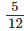The probability of a white   or  a   red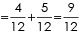or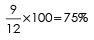When events are not mutually exclusive

The addition theorem studied above is not applicable when the events are not mutually exclusive. In such cases where the events are not mutually exclusive, the probability is : P(A or B) = P(A) + P(B) - P(A and B)

Example 10 :

Two students X and Y work independently on a problem. The probability that A will solve it is 3/4 and the probability that Y will solve it is 2/3. What is the probability that the problem will be solved ?

Solution :

P(A or B)=P(A)+P(B)-P(A and B)

The probability that X will solve the problem is = 3/4 The probability that Y will solve the problem is—2/3 The events are not mutually exclusive as both of them may solve the problem.

Therefore, the probability =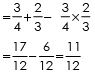Alternatively: The probability that X will solve it and Y fail to solve it = 3/4 x 1/3 = 3/12

∴ Probability that the problem will be solved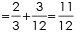Alternatively : The probability that X will fail to solve and will Y solve it

= 1/4 x 2/3 = 2/12

∴ Probability that the problem will be solved =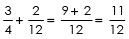Alternatively : The probability that neither X nor Y will solve it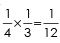Hence, the probability that the problem will be solved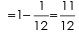Multiplication: When it is desired to estimate the chances of the happening of successive events, the separate probabilities of these successive events are multiplied. If two events A and B are independent, then the probability that both will occur is equal to the product of the respective probabilities. We find the probability of the happening of two or more events in succession.

Symbolically :

P(A and B)=P(A)xP(B)

Example 11 : In two tosses of a fair coin, what are the chances of head in both ?

Solution : Probability of head in first toss = 1/2
Probability of head in the second toss = 1/2
Probability of head in both tosses = 1/2x1/2 -1/4

Example 12 :

The probability that X and Y will be alive ten years hence is 0.5 and 0.8 respectively. What is the probability that both of them will be alive ten years hence ?

Solution : Probability of X being alive ten years hence = 0.5
Probability of Y being alive ten years hence = 0.8
Probability of X and Y both being alive ten years hence =.5x.8=0.4

When events are dependent : If the events are dependent, the probability is conditional. Two events A and B   are dependent ; B occurs only when A is known to have occurred.
P (B|A) means the probability of B given that A has occurred.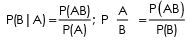Example 13 :

A man want to marry a girl having qualities: White complexion the probability of getting such girl is 1 in 20.
Handsome dowry - the probabihty of getting is 1 in 50. Westernised style - the probability is 1 in 100.
Find out the probability of his getting married to such a girl, who has all the three qualities.

Solution :
The probability of a girl with white complexion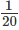or 0.05. The probability of a girl with handsome dowry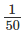= or 0.02.  The probabihty of a girl with westernised style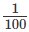= or 0.01. Since the events are independent, the probabihty of simultaneous occurrence of all three qualities =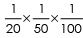= 0.05 x 0.02 x 0.01 = 0.00001

Example 14 :

A university has to select an examiner from a list of 50 persons, 20 of them women and 30 men, 10 of them knowing Hindi and 40 not. 15 of them being teachers and the remaining 35 not. What is the probability of the University selecting a Hindi-knowing women teacher ?

Solution :

Probability of selecting a women = 20/50

Probability  of selecting a teacher =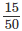Probability of selecting a Hindi-knowing candidate  =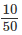Since the events are independent the probabihty of the University selecting a Hindi-knowing woman teacher is :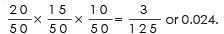Example 15 :

A ball is drawn at random from a box containing 6 red balls, 4 white balls and 5 blue balls. Determine the probability that it is :

(i) Red (ii) white, (iii) Blue, (iv) Not Red and (v) Red or White.

Solution :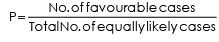(i) Probability of Red 6/15 = or 0.40
(ii)  Probability of white  4/15 = or 0.267
(iii) Probability of Blue 5/15 = A or 0.333
(iv) Probability of not Red 9/15 = or 0.60
(v) Probability of Red and White 10/15 = or 0.667

The document Theorems of Probability - Addition & Multiplication, Business Mathematics and Statistics | Business Mathematics and Statistics - B Com is a part of the B Com Course Business Mathematics and Statistics.
All you need of B Com at this link: B Com

115 videos|142 docs

## FAQs on Theorems of Probability - Addition & Multiplication, Business Mathematics and Statistics - Business Mathematics and Statistics - B Com

 1. What are the Theorems of Probability - Addition & Multiplication?Ans. The Theorems of Probability - Addition & Multiplication are two fundamental theorems in probability theory. The Addition Theorem states that the probability of the union of two events is equal to the sum of their individual probabilities minus the probability of their intersection. The Multiplication Theorem states that the probability of the intersection of two events is equal to the product of their individual probabilities.
 2. How are Theorems of Probability - Addition & Multiplication used in Business Mathematics?Ans. The Theorems of Probability - Addition & Multiplication are used in various aspects of Business Mathematics, such as in analyzing the probability of success or failure of a business venture or investment. They are also used in calculating the expected value, variance, and standard deviation of a random variable in a business context.
 3. What is the importance of understanding Theorems of Probability - Addition & Multiplication in Statistics?Ans. The Theorems of Probability - Addition & Multiplication are essential tools in Statistics, as they provide a framework for calculating the probability of different outcomes in various statistical experiments. Understanding these theorems is crucial in making informed decisions based on statistical data and in drawing meaningful conclusions from statistical analyses.
 4. How do you apply Theorems of Probability - Addition & Multiplication in real-life scenarios?Ans. The Theorems of Probability - Addition & Multiplication can be applied in various real-life scenarios, such as predicting the probability of a stock market crash, estimating the likelihood of a disease outbreak, or calculating the probability of winning a lottery. These theorems can also be used in risk management, insurance, and other business applications.
 5. What are some common mistakes to avoid while applying Theorems of Probability - Addition & Multiplication?Ans. Some common mistakes to avoid while applying Theorems of Probability - Addition & Multiplication include confusing the addition and multiplication theorems, not considering the sample space, and assuming independence between events without proper justification. It is also essential to ensure that the events being considered are mutually exclusive or independent, as the theorems do not hold for non-mutually exclusive or dependent events.

115 videos|142 docsExplore Courses for B Com examSignup to see your scores go up within 7 days! Learn & Practice with 1000+ FREE Notes, Videos & Tests.
10M+ students study on EduRev
Track your progress, build streaks, highlight & save important lessons and more!
Related Searches

,

,

,

,

,

,

,

,

,

,

,

,

,

,

,

,

,

,

,

,

,

,

,

,

;